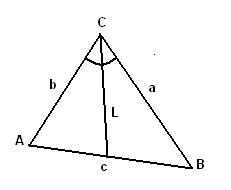# Angle bisector of a triangle

This online calculator computes the length of angle bisector given the lengths of triangle edgesThis online calculator computes the length of the angle bisector given the lengths of triangle edges (see the picture). Triangle vertices are usually named A, B, and C. Triangle edges - a, b, c, where the letter denotes opposite vertex. That is the edge between A and B is named c, between A and C - b, between B and C - a.
The length of С's angle bisector shown on picture is:#### Angle bisector of a triangle

Digits after the decimal point: 2
Length of angle bisector

URL copied to clipboard

#### Similar calculators

PLANETCALC, Angle bisector of a triangle